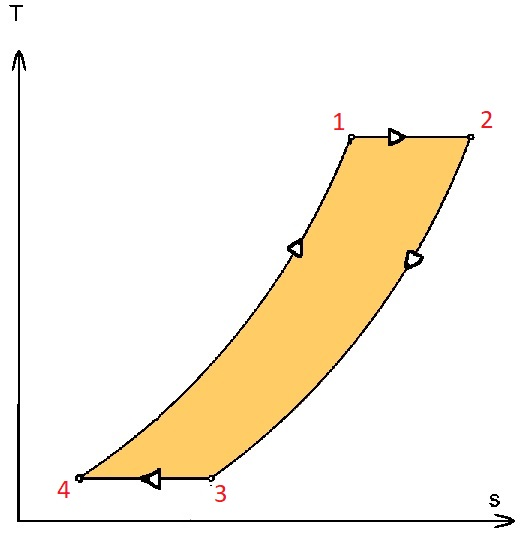# Ericsson cycle | Efficiency, P-V & T-S Diagrams | Heat & Work Table

In this article we will discuss Ericsson cycle | Efficiency, P-V & T-S Diagrams | Heat & Work Table

Ericsson cycle is a thermodynamic cycle upon which an Ericsson Engine works. Ericsson engine is a closed cycle regenerative heat engine. It works on either air or any other gas.

Ericsson cycle was invented by John Ericsson.Ericsson Cycle consists of two isothermal and two constant pressure processes. It is made thermodynamically reversible by the action of a regenerator.

The p-v and T-s diagrams of the Ericsson cycle are shown in the figure. This cycle is used these days in the manufacture of closed-cycle type gas turbines.Ericsson Cycle is comprised of four processes

Process 1-2

It is isothermal heat addition process. Volume of the system increases due to isothermal heat addition. A drop in pressure also happens in this process.

Process 2-3

It is isobaric heat removal process. Both temperature and volume of the system decreases due to isobaric heat removal.

Process 3-4

It is isothermal heat removal process. It is a compression process hence pressure of the system increases and volume decreases.

Process 4-1

It is isobaric heat addition process. Both temperature and volume of the system increases due to isobaric heat addition.

Find the table below which shows heat and work interactions of the Ericsson cycle, along with the change in the internal energy.

 Process Change in Internal Energy Heat Interaction Work Interaction Process 1-2 0 RT1 ln(V2/V1) RT1 ln(V2/V1) Process 2-3 CP(T3-T2) (h3 – h2) P2(V3-V2) Process 3-4 0 RT3 ln(V4/V3) RT3 ln(V4/V3) Process 4-1 CP(T1-T4) (h1 – h4) P1(V1-V4)

Note: Negative value of heat interaction indicates heat rejected by the system and positive value of heat interaction indicates heat added to the system. Positive value of work interaction indicates work done by the system and negative value of work interaction indicates work done on the system.

## Efficiency of Ericsson Cycle

Efficiency of the is the ratio of work output to the heat input.

Work output = [RT1 ln(V2/V1) + CP(T1-T4)] – [CP(T2-T3) + RT3 ln(V3/V4)]

Heat Input = RT1 ln(V2/V1) + CP(T1-T4)

Efficiency = Work Output/Heat Input

After putting values of heat input and work output in the above formula, we get

η = 1 – [(CP(T2-T3) + RT3 ln(V3/V4))/(RT1 ln(V2/V1) + CP(T1-T4))] … (1)

Since, Ericsson cycle is a regenerative cycle hence heat rejected in process 2-3 is used for heat addition in process 4-1. It means CP(T2-T3) gets cancelled by CP(T1-T4) hence, we can replace these values by zero in equation (1).

Also, V3/V4 = V2/V1

Hence, new thermal efficiency (after solving equation (1))

η = 1 – (T3/T1)

Which is equal to Carnot Cycle efficiency.

Tags: